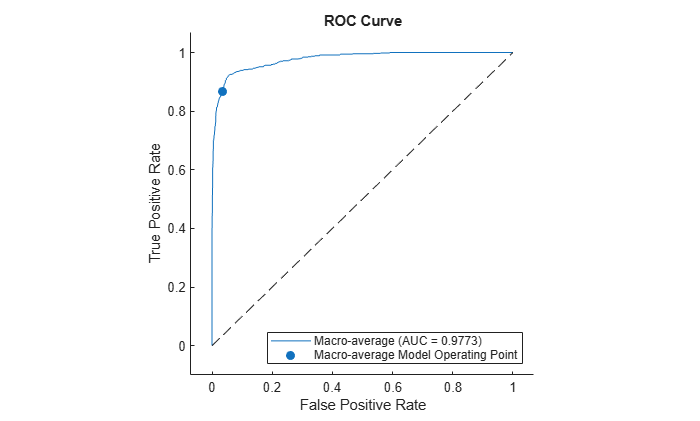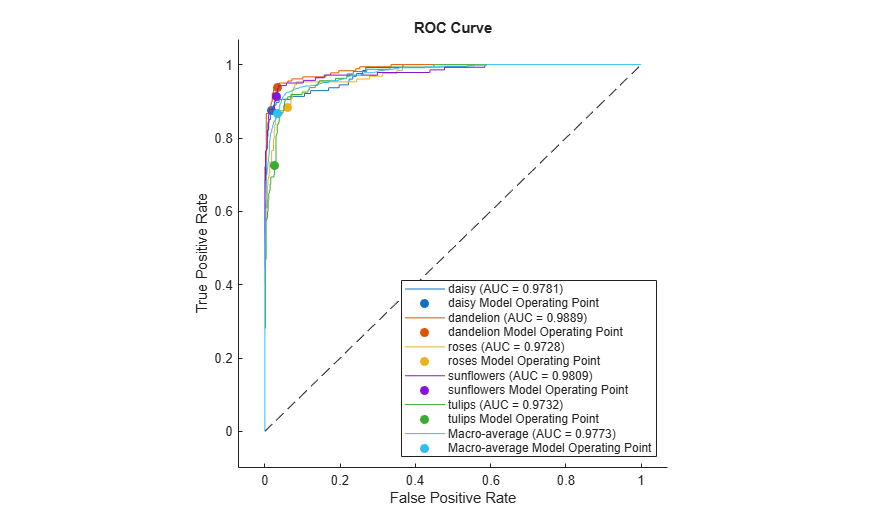# average

Compute performance metrics for average receiver operating characteristic (ROC) curve in multiclass problem

Since R2022b

## Syntax

``[FPR,TPR,Thresholds,AUC] = average(rocObj,type)``

## Description

example

````[FPR,TPR,Thresholds,AUC] = average(rocObj,type)` computes the averages of performance metrics stored in the `rocmetrics` object `rocObj` for a multiclass classification problem using the averaging method specified in `type`. The function returns the average false positive rate (`FPR`) and the average true positive rate (`TPR`) for each threshold value in `Thresholds`. The function also returns `AUC`, the area under the ROC curve composed of `FPR` and `TPR`.```

## Examples

collapse all

Compute the performance metrics for a multiclass classification problem by creating a `rocmetrics` object, and then compute the average values for the metrics by using the `average` function. Plot the average ROC curve using the outputs of `average`.

Load a sample of true labels and the prediction scores for a classification problem. For this example, there are five classes: daisy, dandelion, roses, sunflowers, and tulips. The class names are stored in `classNames`. The scores are the softmax prediction scores generated using the `predict` function. `scores` is an N-by-K array where N is the number of observations and K is the number of classes. The column order of `scores` follows the class order stored in c`lassNames`.

```load('flowersDataResponses.mat') scores = flowersData.scores; trueLabels = flowersData.trueLabels; classNames = flowersData.classNames;```

Create a `rocmetrics` object by using the true labels in `trueLabels` and the classification scores in `scores`. Specify the column order of s`cores` using `classNames`.

`rocObj = rocmetrics(trueLabels,scores,classNames);`

`rocmetrics` computes the FPR and TPR at different thresholds and finds the AUC value for each class.

Compute the average performance metric values, including the FPR and TPR at different thresholds and the AUC value, using the macro-averaging method.

`[FPR,TPR,Thresholds,AUC] = average(rocObj,"macro");`

Plot the average ROC curve and display the average AUC value. Include (0,0) so that the curve starts from the origin `(0,0)`.

```plot([0;FPR],[0;TPR]) xlabel("False Positive Rate") ylabel("True Positive Rate") title("Average ROC Curve") hold on plot([0,1],[0,1],"k--") legend(join(["Macro-average (AUC =",AUC,")"]), ... Location="southeast") axis padded hold off```Alternatively, you can create the average ROC curve by using the `plot` function. Specify `AverageROCType="macro"` to compute the metrics for the average ROC curve using the macro-averaging method.

`plot(rocObj,AverageROCType="macro",ClassNames=[])`## Input Arguments

collapse all

Object evaluating classification performance, specified as a `rocmetrics` object.

Averaging method, specified as `"micro"`, `"macro"`, or `"weighted"`.

• `"micro"` (micro-averaging) — `average` finds the average performance metrics by treating all one-versus-all binary classification problems as one binary classification problem. The function computes the confusion matrix components for the combined binary classification problem, and then computes the average FPR and TPR using the values of the confusion matrix.

• `"macro"` (macro-averaging) — `average` computes the average values for FPR and TPR by averaging the values of all one-versus-all binary classification problems.

• `"weighted"` (weighted macro-averaging) — `average` computes the weighted average values for FPR and TPR using the macro-averaging method and using the prior class probabilities (the `Prior` property of `rocObj`) as weights.

The algorithm type determines the length of the vectors for the output arguments (`FPR`, `TPR`, and `Thresholds`). For more details, see Average of Performance Metrics.

Data Types: `char` | `string`

## Output Arguments

collapse all

Average false positive rates, returned as a numeric vector.

Average true positive rates, returned as a numeric vector.

Thresholds on classification scores at which the function finds each of the average performance metric values (`FPR` and `TPR`), returned as a vector.

Area under the average ROC curve composed of `FPR` and `TPR`, returned as a numeric scalar.

collapse all

### Receiver Operating Characteristic (ROC) Curve

A ROC curve shows the true positive rate versus the false positive rate for different thresholds of classification scores.

The true positive rate and the false positive rate are defined as follows:

• True positive rate (TPR), also known as recall or sensitivity — `TP/(TP+FN)`, where TP is the number of true positives and FN is the number of false negatives

• False positive rate (FPR), also known as fallout or 1-specificity — `FP/(TN+FP)`, where FP is the number of false positives and TN is the number of true negatives

Each point on a ROC curve corresponds to a pair of TPR and FPR values for a specific threshold value. You can find different pairs of TPR and FPR values by varying the threshold value, and then create a ROC curve using the pairs. For each class, `rocmetrics` uses all distinct adjusted score values as threshold values to create a ROC curve.

For a multiclass classification problem, `rocmetrics` formulates a set of one-versus-all binary classification problems to have one binary problem for each class, and finds a ROC curve for each class using the corresponding binary problem. Each binary problem assumes one class as positive and the rest as negative.

For a binary classification problem, if you specify the classification scores as a matrix, `rocmetrics` formulates two one-versus-all binary classification problems. Each of these problems treats one class as a positive class and the other class as a negative class, and `rocmetrics` finds two ROC curves. Use one of the curves to evaluate the binary classification problem.

For more details, see ROC Curve and Performance Metrics.

### Area Under ROC Curve (AUC)

The area under a ROC curve (AUC) corresponds to the integral of a ROC curve (TPR values) with respect to FPR from `FPR` = `0` to `FPR` = `1`.

The AUC provides an aggregate performance measure across all possible thresholds. The AUC values are in the range `0` to `1`, and larger AUC values indicate better classifier performance.

### One-Versus-All (OVA) Coding Design

The one-versus-all (OVA) coding design reduces a multiclass classification problem to a set of binary classification problems. In this coding design, each binary classification treats one class as positive and the rest of the classes as negative. `rocmetrics` uses the OVA coding design for multiclass classification and evaluates the performance on each class by using the binary classification that the class is positive.

For example, the OVA coding design for three classes formulates three binary classifications:

Each row corresponds to a class, and each column corresponds to a binary classification problem. The first binary classification assumes that class 1 is a positive class and the rest of the classes are negative. `rocmetrics` evaluates the performance on the first class by using the first binary classification problem.

## Algorithms

collapse all

### Adjusted Scores for Multiclass Classification Problem

For each class, `rocmetrics` adjusts the classification scores (input argument `Scores` of `rocmetrics`) relative to the scores for the rest of the classes if you specify `Scores` as a matrix. Specifically, the adjusted score for a class given an observation is the difference between the score for the class and the maximum value of the scores for the rest of the classes.

For example, if you have [s1,s2,s3] in a row of `Scores` for a classification problem with three classes, the adjusted score values are [s1-`max`(s2,s3),s2-`max`(s1,s3),s3-`max`(s1,s2)].

`rocmetrics` computes the performance metrics using the adjusted score values for each class.

For a binary classification problem, you can specify `Scores` as a two-column matrix or a column vector. Using a two-column matrix is a simpler option because the `predict` function of a classification object returns classification scores as a matrix, which you can pass to `rocmetrics`. If you pass scores in a two-column matrix, `rocmetrics` adjusts scores in the same way that it adjusts scores for multiclass classification, and it computes performance metrics for both classes. You can use the metric values for one of the two classes to evaluate the binary classification problem. The metric values for a class returned by `rocmetrics` when you pass a two-column matrix are equivalent to the metric values returned by `rocmetrics` when you specify classification scores for the class as a column vector.

## Alternative Functionality

• You can use the `plot` function to create the average ROC curve. The function returns a `ROCCurve` object containing the `XData`, `YData`, `Thresholds`, and `AUC` properties, which correspond to the output arguments `FPR`, `TPR`, `Thresholds`, and `AUC` of the `average` function, respectively. For an example, see Plot ROC Curve.

 Sebastiani, Fabrizio. "Machine Learning in Automated Text Categorization." ACM Computing Surveys 34, no. 1 (March 2002): 1–47.